# Knowing Different Kinds of Triangle

In this worksheet, students identify different types of triangles classified by their sides, by their angles or by a combination of both sides and angles.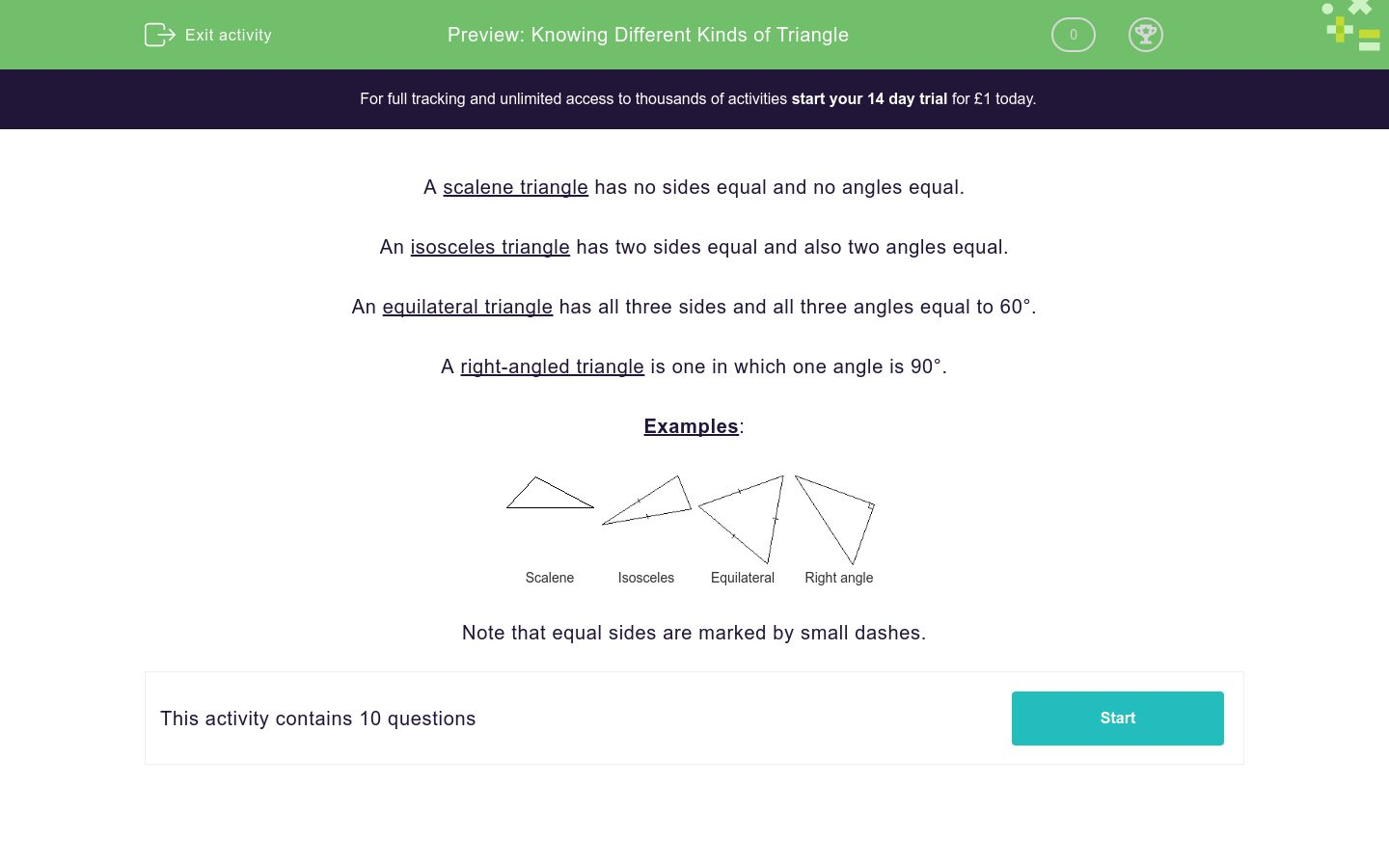Key stage:  KS 3

Curriculum topic:   Geometry and Measures

Curriculum subtopic:   Derive and Illustrate Properties of Plane Figures

Difficulty level:### QUESTION 1 of 10

A scalene triangle has no sides equal and no angles equal.

An isosceles triangle has two sides equal and also two angles equal.

An equilateral triangle has all three sides and all three angles equal to 60°.

A right-angled triangle is one in which one angle is 90°.

Examples: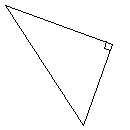Scalene Isosceles Equilateral Right angle

Note that equal sides are marked by small dashes.

Identify the type of triangle shown below.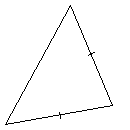Equilateral

Isosceles

Scalene

Right-angled

Identify the type of triangle shown below.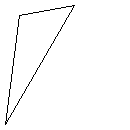Equilateral

Isosceles

Scalene

Right-angled

Identify the type of triangle shown below.Equilateral

Isosceles

Scalene

Right-angled

Identify the type of triangle shown below.Right-angled

Scalene

Isosceles

Equilateral

Identify the type of triangle shown below.Right-angled

Scalene

Isosceles

Equilateral

Identify the type of triangle shown below.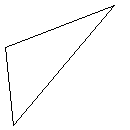Equilateral

Isosceles

Scalene

Right-angled

Identify the type of triangle shown below.Equilateral

Isosceles

Scalene

Right-angled

Identify the type of triangle shown below.Equilateral

Isosceles

Scalene

Right-angled

Identify the type of triangle shown below.Equilateral

Isosceles

Scalene

Right-angled

Identify the type of triangle shown below.Scaleen

Scaly

Scalene

Scaline

• Question 1

Identify the type of triangle shown below.Isosceles
EDDIE SAYS
The litlle dashes on the sides of the triangle mean that those sides are equal. As this triangle has two equal sides it must be an isosceles.
• Question 2

Identify the type of triangle shown below.Scalene
EDDIE SAYS
This triangle has no dashes on the sides so all the sides are different lengths. This means this triangle is a scalene triangle.
• Question 3

Identify the type of triangle shown below.Right-angled
EDDIE SAYS
The triangle has a little square box for one angle. This means the triangle is right angled.
• Question 4

Identify the type of triangle shown below.Isosceles
EDDIE SAYS
The litlle dashes on the sides of the triangle mean that those sides are equal. As this triangle has two equal sides it must be an isosceles.
• Question 5

Identify the type of triangle shown below.Equilateral
EDDIE SAYS
The little dashes on all three sides means that all the sides are equal. This must be an equilateral triangle.
• Question 6

Identify the type of triangle shown below.Scalene
EDDIE SAYS
This triangle has no dashes on the sides so all the sides are different lengths. This means this triangle is a scalene triangle.
• Question 7

Identify the type of triangle shown below.Scalene
EDDIE SAYS
This triangle has no dashes on the sides so all the sides are different lengths. This means this triangle is a scalene triangle.
• Question 8

Identify the type of triangle shown below.Right-angled
EDDIE SAYS
The triangle has a little square box for one angle. This means the triangle is right angled.
• Question 9

Identify the type of triangle shown below.Equilateral
EDDIE SAYS
The little dashes on all three sides means that all the sides are equal. This must be an equilateral triangle.
• Question 10

Identify the type of triangle shown below.Scalene
EDDIE SAYS
This triangle has no dashes on the sides so all the sides are different lengths. This means this triangle is a scalene triangle.
---- OR ----

Sign up for a £1 trial so you can track and measure your child's progress on this activity.

### What is EdPlace?

We're your National Curriculum aligned online education content provider helping each child succeed in English, maths and science from year 1 to GCSE. With an EdPlace account you’ll be able to track and measure progress, helping each child achieve their best. We build confidence and attainment by personalising each child’s learning at a level that suits them.

Get started